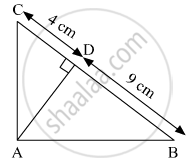# Some Question and Their Alternative Answer Are Given. Altitude on the Hypotenuse of a Right Angled Triangle Divides It in Two Parts of Lengths 4 Cm and 9 Cm. Find the Length of the Altitude. - Geometry

MCQ

Some question and their alternative answer are given. Select the correct alternative.

Altitude on the hypotenuse of a right angled triangle divides it in two parts of lengths 4 cm and 9 cm. Find the length of the altitude.

#### Options

• 9 cm

• 4 cm

• 6 cm

• $2\sqrt{6}$ cm

#### SolutionWe know that,
In a right angled triangle, the perpendicular segment to the hypotenuse from the opposite vertex, is the geometric mean of the segments into which the hypotenuse is divided.

$\therefore {AD}^2 = CD \times DB$
$= 4 \times 9$
$= 36$
$\Rightarrow AD = 6 cm$

Hence, the  correct option is 6 cm.

Concept: Apollonius Theorem
Is there an error in this question or solution?

#### APPEARS IN

Balbharati Mathematics 2 Geometry 10th Standard SSC Maharashtra State Board
Chapter 2 Pythagoras Theorem
Problem Set 2 | Q 1.6 | Page 43